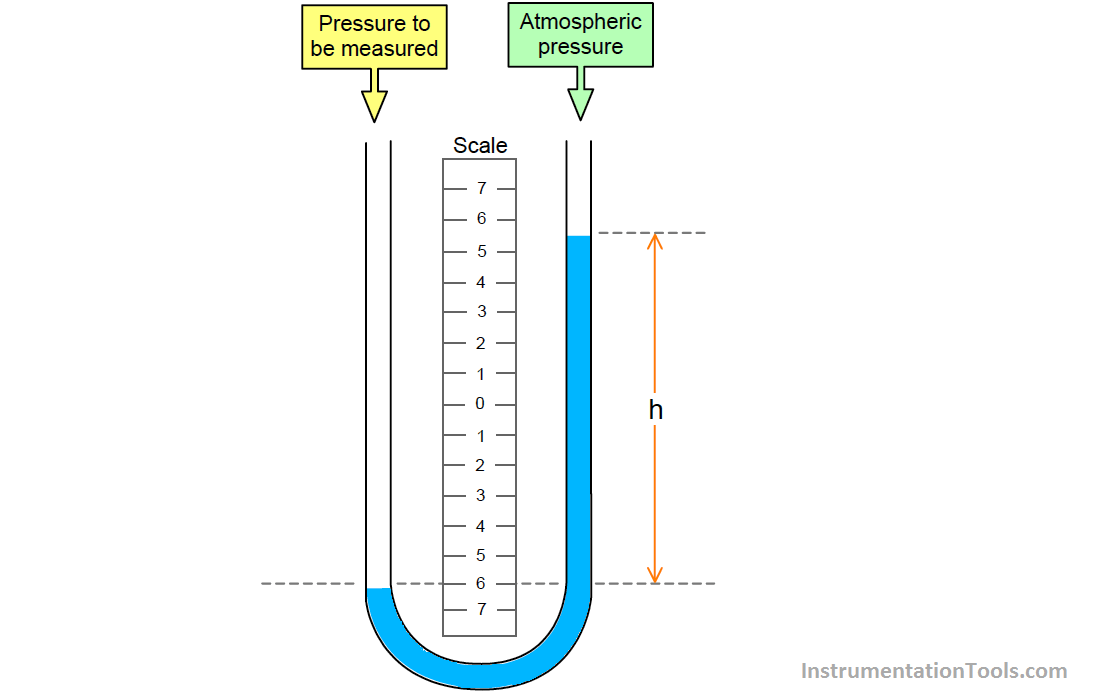# U-tube Manometer Principle

The simplest form of manometer consists of a U-shaped glass tube containing liquid. It is used to measure gauge pressure and are the primary instruments used in the workshop for calibration.The principle of the manometer is that the pressure to be measured is applied to one side of the tube producing a movement of liquid, as shown in figure above. It can be seen that the level of the filling liquid in the leg where the pressure is applied, i.e. the left leg of the tube, has dropped, while that in the right hand leg as risen. A scale is fitted between the tubes to enable us to measure this displacement.

Let us assume that the pressure we are measuring and have applied to the left hand side of the manometer is of constant value. The liquid will only stop moving when the pressure exerted by the column of liquid, H is sufficient to balance the pressure applied to the left side of the manometer, i.e. when the head pressure produced by column ” H ” is equal to the pressure to be measured.

Knowing the length of the column of the liquid, H, and density of the filling liquid, we can calculate the value of the applied pressure.

The applied Pressure = ρ × g × h

y suitable choice of filling liquid, various low ranges of gauge pressure can be measured from about 500 Pa to 1.5 bar.

Typical filling liquids commonly used in manometers and their densities.

1. Water ( ρ = 1000 kg m-3 )
2. Oil ( ρ can be between 800 and 950 kg m-3 )
3.  Mercury ( ρ = 13560 kg m-3 )

Credits : N Asyiddin